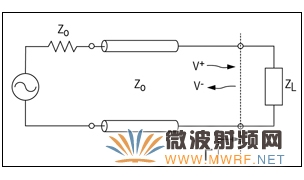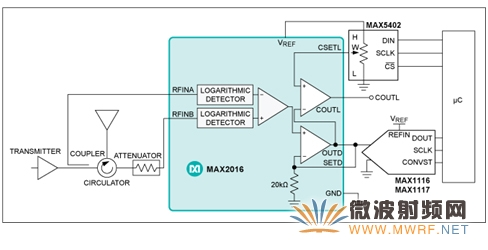## 测量电压驻波比(VSWR)量化传输线的阻抗失配

2022-11-10 13:53发布

SWR反映了入射波与反射波的比率，SWR越高表明传输线效率越低、反射能量越大，可能导致发射机损坏，降低发射效率。由于SWR通常用电压比表示，也称为电压驻波比(VSWR)。

VSWR和系统效率

 Γ = V-/V+ (Eq. 1)

 VSWR = (1 + |Γ|)/(1 – |Γ|) (Eq. 2)RLIN = 20log10|S11| dB (Eq. 3) RLOUT = 20log10|S22| dB (Eq. 4)

 Γ = (ZL - ZO)/(ZL + ZO) (Eq. 5)

VSWR也可以用ZL和ZO表示。把式5代入式2，可以得到：
VSWR = [1 + |(ZL - ZO)/(ZL + ZO)|]/[1 - |(ZL - ZO)/(ZL + ZO)|] = (ZL + ZO + |ZL - ZO|)/(ZL + ZO - |ZL - ZO|)

 VSWR = (ZL + ZO + ZL - ZO)/(ZL + ZO - ZL + ZO) = ZL/ZO (Eq. 6)

 VSWR = (ZL + ZO + ZO - ZL)/(ZL + ZO - ZO + ZL) = ZO/ZL (Eq. 7)

VSWR的定义是来自于传输线自身产生的驻波：

 VSWR = |VMAX|/|VMIN| (Eq. 8)

 VMAX = V+ + V- (Eq. 9)

 VMIN = V+ - V- (Eq. 10)

 VSWR = |VMAX|/|VMIN| = (V+ + V-)/(V+ - V-) (Eq. 11)

 VSWR = V+(1 + |Γ|)/(V+(1 - |Γ|) = (1 + |Γ|)/(1 – |Γ|) (Eq. 12)

VSWR检测系统

MAX2016为双通道对数检测器/控制器，它与环行器和衰减器配套使用，用于监测天线的VSWR/回波损耗。MAX2016可输出两路功率检测器的差。